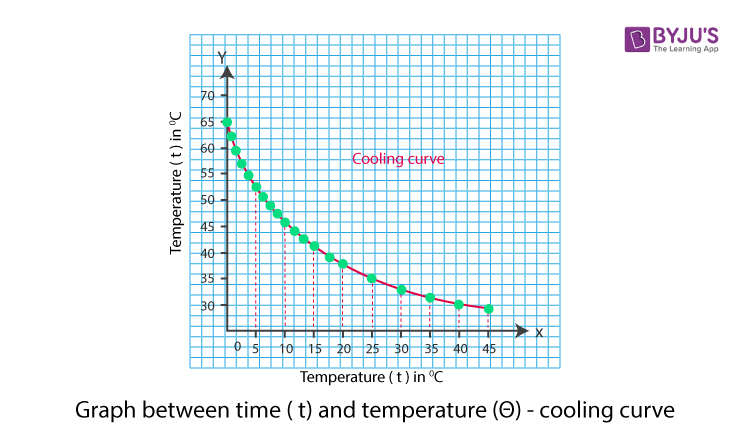# To Study the Relationship between the Temperature of a Hot Body and Time by Plotting a Cooling Curve

According to Newton’s law of cooling, the rate of cooling of a body is directly proportional to the difference in temperature of the body and the surrounding provided the difference in the temperature shouldn’t exceed 30°C. In this experiment, we will be validating the law by studying the relationship between the temperature of a hot body and time by plotting the cooling curve.

## Aim

To study the relationship between the temperature of a hot body and time by plotting the cooling curve.

## Apparatus and Materials Required

• Newton’s law of cooling apparatus (copper calorimeter with a wooden lid having two holes for inserting a thermometer and a stirrer and an open double – walled vessel)
• Two thermometers
• Stop clock
• Heater
• Burner
• Water
• Clamp Stand
• Two rubber stoppers with holes
• Beaker

## Theory

Newton’s law of cooling states that the rate of cooling of a body is directly proportional to the temperature difference between the body and the surrounding, provided the temperature difference is small.

Mathematically, it can be expressed as follows:

$$\begin{array}{l}\frac{dQ}{dt}\propto (T_1-T_2)\end{array}$$

For a body of mass m, specific heat c, with a temperature T kept in the surrounding of temperature T0, the heat energy is given as follows:

Rate of cooling,

$$\begin{array}{l}\frac{dQ}{dt}=ms\frac{dT}{dt}\end{array}$$

Hence,

$$\begin{array}{l}ms\frac{dT}{dt}\propto (T-T_0)\end{array}$$

ms is a constant,

$$\begin{array}{l}\frac{dT}{dt}\propto (T-T_0)\end{array}$$

From the above relation, it is clear that as time increases, T decreases, (TT0) decreases, as result the fall of temperature (dT/dt) must also decrease.

## Procedure

1. Fill the space between the double wall of the enclosure with water and keep it on top of a table.
2. Fill two-thirds of the calorimeter with water heated to about 80 °C.
3. Suspend the calorimeter inside the enclosure with a thermometer in it. Cover it with a wooden lid with a hole in the middle.
4. Suspend a thermometer from the clamp and stand into the enclosure water and the other thermometer in calorimeter water.
5. Note the least count of thermometers.
6. Set the stop clock to zero and note down its least count.
7. Note the temperature T0 of water in the enclosure.
8. Start stirring the water in calorimeter so that it cools uniformly.
9. When the calorimeter has convenient temperature reading, note it down and start and stop the clock watch
10. Continue stirring and note the temperature after every few minutes. The temperature falls quickly in the first few minutes
11. Note down the enclosure water temperature every five minutes.
12. When the temperature fall becomes slow, note down the temperature at an interval of two minutes for ten minutes and then an interval of 5 minutes.
13. Stop when the fall of temperature becomes very slow.
14. Record your observation as given in the table below

## Observation

The Least count of enclosure water thermometer = _____ °C

The Least count of calorimeter water thermometer = _____ °C

The Least count of stop clock watch = _____ s

 S.No of observations Time For Cooling t Temperature of water in calorimeter (T °C) Temperature of water in enclosure (T0 °C) Difference in temperature T – T0 °C

### Calculation

1. The temperature of water in the enclosure will be found to be the same. If not, then take its mean.
2. Find the temperature difference (TT0)
3. Plot a graph between temperature T and time t as shown below. This graph is known as the cooling curve of the liquid.## Result

The temperature is seen to fall quickly in the beginning and then the difference in temperature slowly decreases. This is in agreement with Newton’s law of cooling.

## Viva Voce

1. Why is water used as a cooling and heating agent?

Answer: Water has high specific heat capacity because of which it absorbs more quantity of heat than others.

2. State Newton’s law of cooling.

Answer: Newton’s law of cooling states that the rate of change of temperature of a body is directly proportional to the difference between its own temperature and the temperature of its Surrounding.

3. Why does a baby need more wrapping of woollen clothes than an adult?

Answer: Mass and the rate of fall of temperature are inversely proportional to each other. As the mass of a baby is lesser than an adult man, the baby loses a larger amount of heat than a grownup adult. Hence, a baby needs more wrapping.

4. Why is it easy to heat a hot pudding in a large plate than it is to heat it in a bowl?

Answer: The rate of cooling is directly proportional to the volume. Hence, a large plate cools faster than a bowl.

5. Define specific heat capacity.

Answer: The heat required to raise the temperature of unit mass by 1oC is known as the specific heat capacity

Stay tuned with BYJU’S to get the latest notification on CBSE along with CBSE syllabus, sample papers, marking scheme and more.

Test Your Knowledge On Relationship Between Temperature Of Hot Body And Time By Plotting Cooling Curve!